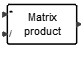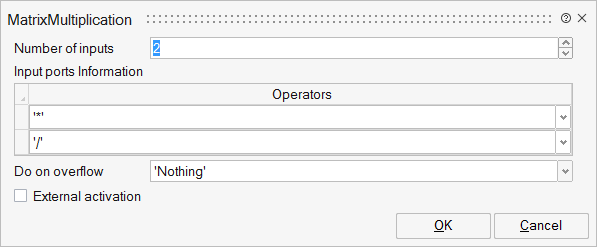# MatrixMultiplication

This block computes the matrix multiplication or division of input matrices. For the multiplication, regular matrix multiplication size rules apply: the number of rows of a matrix must be equal to the number of columns of the next matrix. For the division, the matrix must be square. This block supports all the integer, real and complex data types.MatrixOperations

## Description

This block computes the matrix multiplication or division of input matrices. For the multiplication, regular matrix multiplication size rules apply: the number of rows of a matrix must be equal to the number of columns of the next matrix. For the division, the matrix must be square. This block supports all the integer, real and complex data types.

## ParametersNameLabelDescriptionData TypeValid Values

nin

Number of inputs

Number

in_ports

Input ports Information

Structure

in_ports/sgn

Operators

* indicates the corresponding input is to be multiplied with other inputs.
/ indicates the corresponding input is inversed and multiplied with other inputs
H indicates the corresponding input is conjugate-transposed and multiplied with other inputs.

Cell of strings

'*'
'/'
'H'

overflow

Do on overflow

String

'Nothing'
'Saturate'
'Error'

externalActivation

External activation

Specifies whether the block receives an external activation or inherits its activation through its regular input ports. When External Activation is selected, an additional activation port is added to the block. By default, external activation is not selected.

Number

0
1

## Ports

NameTypeDescriptionIO TypeNumber

Port 1

explicit

output

1

Port 2

explicit

input

nin

Port 3

activation

input

externalActivation

NameValueDescription

always active

no

direct-feedthrough

yes

zero-crossing

no

mode

no

continuous-time state

no

discrete-time state

no